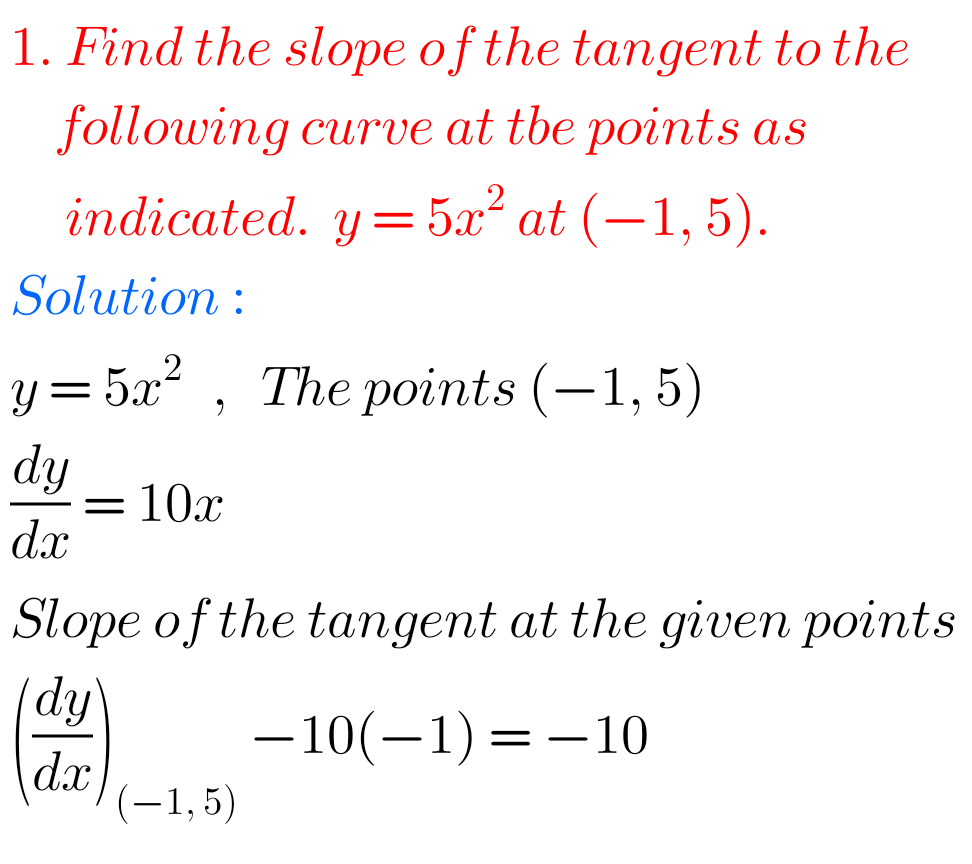## Tangent and Normal,Intermediate first year mathematics 1B chapter 10.2 problems with solutions

TANGENT AND NORMAL Solutions, Inter first year mathematics 1B problems with solutions Mathematics Intermediate1B solutions for some problems for Tangents and Normal. These solutions are very simple to understand. Study the lesson Applications of Derivatives very well. Observe the example problems and solutions given in the textbook. Applications of Derivatives Exercise 10(a) Exercise 10(b) Exercise …